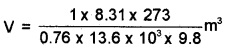# Plus One Physics Chapter Wise Questions and Answers Chapter 12 Thermodynamics

Students can Download Chapter 12 Thermodynamics Questions and Answers, Plus One Physics Chapter Wise Questions and Answers helps you to revise the complete Kerala State Syllabus and score more marks in your examinations.

## Kerala Plus One Physics Chapter Wise Questions and Answers Chapter 12 Thermodynamics

### Plus One Physics Thermodynamics One Mark Questions and Answers

Question 1.
If the pressure and the volume of certain quantity of ideal gas are halved, then its temperature
(a) is doubled
(b) becomes one-fourth
(c) remains constant
(d) is halved
(b) becomes one-fourth
According to ideal gas law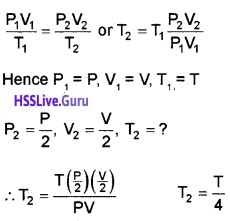Question 2.
The ideal gas equation connecting pressure (P), volume (V) and absolute temperature (T) is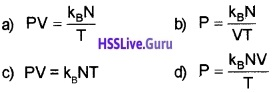where kB is the Boltzmann constant and N is the number of molecules.
c) PV = kBNT
According to ideal gas equation,
PV = NkBT.

Question 3.
Which of the following laws of thermodynamics forms the basis for the definition of temperature?
(a) First law
(b) Zeroth law
(c) Second law
(d) Third’law
(b) Zeroth law
The Zeroth law of thermodynamics gives the definition of temperature.

Question 4.
Which of the following is a true statement?
(a) The total entropy of thermally interacting systems is conserved
(b) Carnot engine has 100% efficiency
(c) Total entropy does not change in a reversible process.
(d) Total entropy in an irreversible process can either increase or decrease.
(c) Total entropy does not change in a reversible process.Question 5.
In a given process of an ideal gas, dW = 0 and dQ < 0. Then for the gas
(a) the temperature will decrease,
(b) the volume will increase
(c) the pressure will remain constant
(d) the temperature will increase
(a) From first law of thermodynamics
dQ = dU + dW
dQ = dU (if dW = 0)
Since, dQ < 0
dU < 0
or Ufinal < Uintial
Hence, temperature will decrease.

Question 6.
An electric fan is switched on in a closed room will the air of the room be cooled?
No. Infact speed of air molecules will increase and this results in increase in temperature.

Question 7.
When an iron nail is hammered, if becomes hot. Why?
The kinetic energy of hammer gets converted in to heat energy which increases temperature of iron nail.

Question 8.
Identify the thermodynamic process in which temperature of system may increase even when no heat is supplied to the system.Question 9.
Which thermodynamic variable is defined by first law of thermodynamics?
Internal energy

Question 10.
The door of an operating refrigerator kept open in a closed room. Will it make the room cool?
No. The room will be slightly warmed.

### Plus One Physics Thermodynamics Two Mark Questions and Answers

Question 1.
A Carnot engine working between 300K and 600K has a work output of 800J per cycle. Find the amount of energy consumed per cycle

• 800J
• 400J
• 1600J
• 1200J
• 3200J

Efficiency of carrots engineEfficiency also can be written as∴ input power = 1600 J.Question 2.
A carnote’s engine is made to work between 200°C and 0°C first and then between 0°C and minus 200°C. Compare the values of efficiencies in the two cases.
Case -1: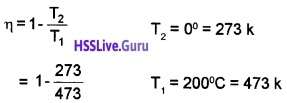= 1 – 0.577
= 0.42 = 42%
Case – 2:
η = 1 – $$\frac{73}{473}$$
T2 = -200 = 73k
= 1 – 0.26 = 0.73
= 73%
T1 = 0 = 273k.

Question 3.
Match the following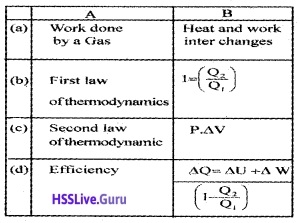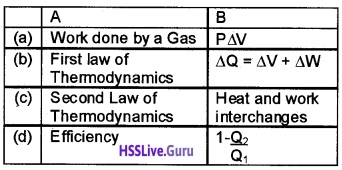Question 4.
What is the value of specific heat capacity of gas in

1. Isothermal process

1. Infinite
2. Zero

Question 5.
Is the function of refrigerator against the second law of thermodynamics? Explain.
No. The refrigerator transfers heat from the inside space to outer atmosphere, at the expense of external work supplied by the compresser fed by electric supply.

### Plus One Physics Thermodynamics Three Mark Questions and Answers

Question 1.
Heat is supplied to a system, but its internal energy does not increase

1. Which process is involved in this case? (1)
2. Obtain an expression for the work done in the above process (2)

1. Isothermal process.

2. Work done by adiabatic process
Let an ideal gas undergoes adiabatic charge from (P1, V1, T1) to (P2, V2, T2). The equation for adiabatic charge is
PVγ = constant = k
ie; P1V1γ = P2V2γ = k _____(a)
The work done by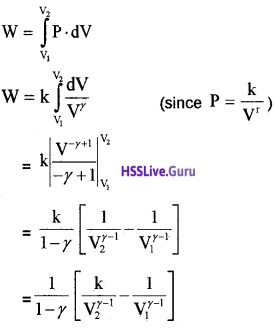from equation (a) P1V1γ = P2V2γ = k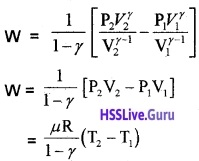Substituting ideal gas equation.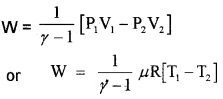Question 2.
A heat engine is a device which effectively converts heat energy into mechanical energy.

1. State the law which describes this principle. (1)
2. Derive an expression for the efficiency of a Carnot engine. (2)

1. Kelvin – Plank statement:
No process is possible whose sole result is the absorption of heat from a reservoir and complete conversion of heat into work.

Clausius statement:
No process is possible whose sole result is the transfer of heat from a colder object to hotter object.

2. Carnot’s cycle:
The Carnot cycle consists of two isothermal processes and two adiabatic processes.Let the working substance in Carnot’s engine be the ideal gas.
Step 1: The gas absorbs heat Q1 from hot reservoir at T1 and undergoes isothermal expansion from (P1, V1, T1) to (P2, V2, T1).

Step 2: Gas undergoes adiabatic expansion from (P2, V2, T1) to (P3, V3, T2)

Step 3: The gas release heat Q2 to cold reservoir at T2, by isothermal compression from (P3, V3, T2) to (P4, V4, T2).

Step 4: To take gas into initial state, work is done on gas adiabatically [(P4, V4, T2) to (P1, V1, T1)]. Efficiency of Carnot’s engine: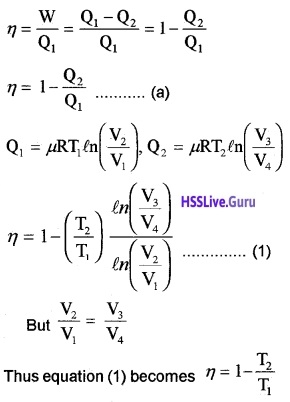Question 3.
A thermo flask contains coffee. It is violently shaken. Considering the coffee as a system:

1. does its temperature rise?
2. has heat been added to its?
3. has internal energy changed?

1. Yes
2. Heat is added to coffee
3. Internal energy is changed
4. When we shake, the mechanical energy is added to the liquid contained in a flask.

Question 4.
It is predicted by the meteorologists that global warming will result in the flooding of oceans due to the melting of ice caps on the earth.

1. Name the thermodynamic process involved in the melting of ice.
2. Determine the heat energy required to melt 5kg of ice completely at 0°C. Latent heat of fusion of water is 3336 × 103 J Kg-1.
3. During the melting process, what change will occur to its internal energy?

1. Isothermal process
2. ∆Q = mL = 5 × 33.36 × 105 = 16.68 × 106J
3. Internal energy increasesQuestion 5.
Water is heated in an open vessel.

1. This process is
• isothermal
• isobaric
• isochoric
2. Which law of thermodynamics is suitable to explain the transfer of heat here?
3. Draw the heat-temperature graph of ice below 0°C heated up to steam above 100°C.

1. Isobaric
2. First law of thermodynamics
3.Question 6.

1. The molar heat capacity of oxygen at constant volume is 20J mol-1K-1. What do you mean by molar heat capacity at constant volume (CV)?
2. The difference between CP and CV is always a constant. Give a mathematical proof.

1. Molar specific heat at constant volume is the heat required to raise the temperature of I mol substance by IK at constant volume.

2. According to 1st law of thermodynamics
∆Q = ∆U + PAV
If ∆Q heat is absorbed at constant volume (∆V = 0).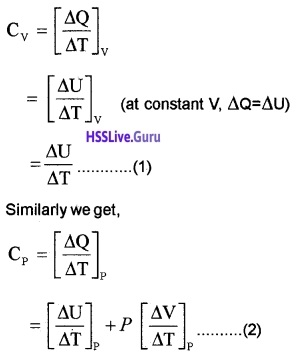From ideal gas equation for one mole PV = RT. Differentiating w.r.t. temperature (at constant pressure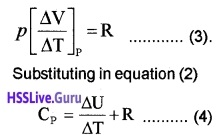Equation (4) – Equation (1), we get
CP – CV = R.Question 7.
A heat engine is a device which converts heat energy into work.

1. What is the working substance in an ideal heat engine.
2. A Carnot engine is working between in melting point and steam point. What is its efficiency?

1. Ideal gas.

2. T2 = 0°C = 273K
T1 = 100°C = 373K
Efficiency η = I – $$\frac{T_{2}}{T_{1}}=I-\frac{273}{373}$$
η = 0.27
η = 27%.

Question 8.
A gas is taken in a cylinder. The walls of the cylinder is insulated from the surroundings

1. The gas in a cylinder is suddenly compressed. Which thermo dynamic process involves in this statement. Explain the thermo dynamic process.
2. If gas is suddenly compressed to 1/4th of its, original volume. Calculate the rise in temperature. The initial temperature was 27°C and γ = 1.5.

1. Adiabatic. A thermo dynamic process in which no heat enters or leaves the system.

2. T. = 27°C = 27 + 273 = 300k= 300 × 41.5 – 1
= 300 × 41/2
= 600K
rise in temperature = 600K – 300K = 300K.

Question 9.
Raju brought a motor pump from a shop. The efficiency of the motor pump is printed on the label as 60%.

1. The efficiency of a water pump is 60%. What is . meant by this?
2. Can you design an engine of 100% efficiency? Justify your answer.

1. Efficiency means that, 60% of the total energy received is converted into useful work.

2. The efficiency of heat engine η = 1 – $$\frac{T_{2}}{T_{1}}$$. The efficiency will be 100% if T2 = OK, both those conditions cannot be attained practically. So art engine can’t have 100% efficiency.Question 10.
Categorise into reversible and irreversible process

1. Waterfall
2. rusting of iron
3. electrolysis
4. slow compression
5. diffusion of gas
6. melting of ice
7. dissolving NaCl in water
8. flow of heat from hot body to cold body
9. slow compression of spring
10. heat produced by friction.

Reversible process → (3), (4), (6) and (9)
Irreversible process → (1), (2), (5), (7), (8) and (10)

### Plus One Physics Thermodynamics NCERT Questions and Answers

Question 1.
A geyser heats water flowing at the rate of 3.0 litres per minute from 27°C to 77°C. If the geyser operates on a gas burner, what is the rate of consumption of the fuel if its heat of combustion is 4.0 × 104 Jg-1?
Volume of water heated = 3.0 litre per minute
mass of water heated, m = 3000 g per minute
increase in temperature, ∆T = 77°C – 27°C = 50°C
specific heat of water, c= 4.2Jg-1 °C-1
amount of heat used, Q = mc ∆T
or Q = 3000 g min-1 × 4.2Jg-1
°C-1 × 50°C
= 63 × 104J min-1
rate of combustion of fuel = $$\frac{63 \times 10^{4} \mathrm{Jmin}^{-1}}{4.0 \times 10^{4} \mathrm{Jg}^{-1}}$$
= 15.75gmin-1.Question 2.
What amount of heat must be supplied to 2.0 x 10-2 kg of nitrogen (at room temperature) to raise its temperature by 45°C at constant pressure? (Molecular mass of N2 = 28, R = 8.3 J mol-1 K-1).
m = 2 × 103 kg-1 = 20g,
∆T = 45°C, M = 28
R = 8.3 J mol-1 K-1, M = 28
Number of moles, n = $$\frac{m}{M}=\frac{20}{28}=\frac{5}{7}$$
Since nitrogen is diatomic,
∴ CP = $$\frac{7}{2}$$
R = $$\frac{7}{2}$$ × 8.3 J mol-1K-1
Now, ∆Q = nCP ∆T
= $$\frac{5}{2}$$ × $$\frac{7}{2}$$ × 8.3 × 45J = 933.75J.

Question 3.
A cylinder with a movable piston contains 3 moles of hydrogen at standard temperature and pressure. The walls of the cylinder are made of a heat insulator, and the piston is insulated by having a pile of sand on it. By what factor does the pressure of the gas increase if the gas, is compressed to half its original volume?
P2V2γ = P1V1γ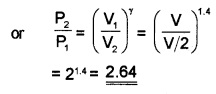Question 4.
A steam engine delivers 5.4 × 108 J of work per minute and services 3.6 × 109J of heat per minute from its boiler. What is the efficiency of the engine? How much heat is wasted per minute?
Work done per minute, output = 5.4 × 108J
Heat absorbed per minute, input = 3.6 × 109J
Efficiency, η = $$\frac{5.4 \times 10^{8}}{3.6 \times 10^{9}}$$ = 0.15
%η = 0.15 × 100 = 15
Heat energy wasted/ minute = Heat energy absorbed/minute – Useful work done/minute
= 3.6 × 109 – 5.4 × 108
= (3.6 – 0.54) × 109 = 3.06 × 109J.Question 5.
An electric heater supplies heat to a system at a rate of 100W. If system performs work at a rate of 75 joule per second, at what rate is the internal energy increasing?
Heat supplied, ∆Q = 100W = 10OJs-1
Useful work done, ∆W = 75J s-1
Using first law of thermodynamics
∆Q = ∆U+ ∆W
∆U = ∆Q – ∆W
= 100Js-1 – 75Js-1 = 25Js-1.

Question 6.
A refrigerator is to maintain eatables kept inside at 9°C. If room temperature is 36°C, calculate the coefficient of performance.
Coefficient of performance = $$\frac{T_{2}}{T_{1}-T_{2}}$$PV = µRT or V = $$\frac{\mu \mathrm{RT}}{\mathrm{P}}$$